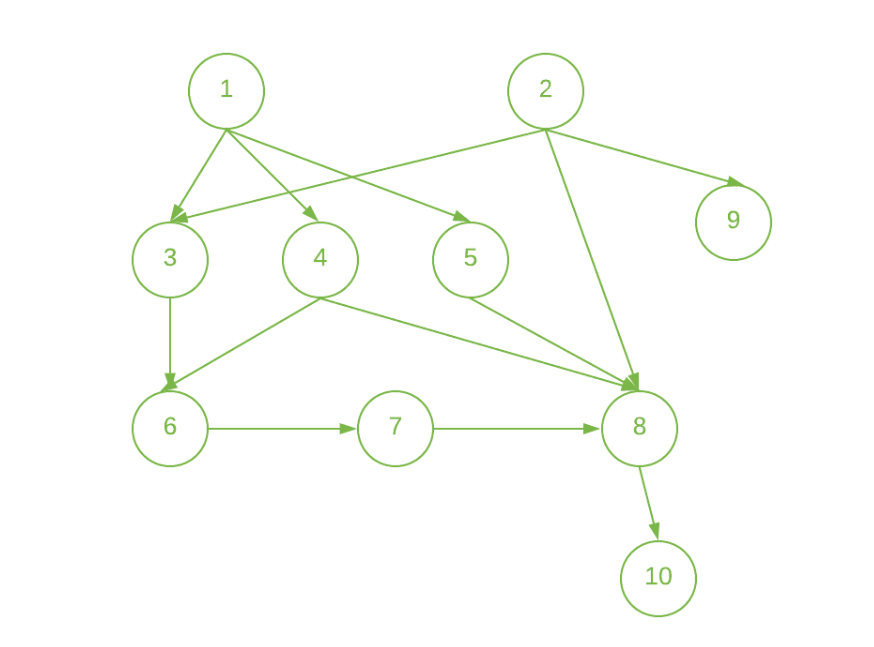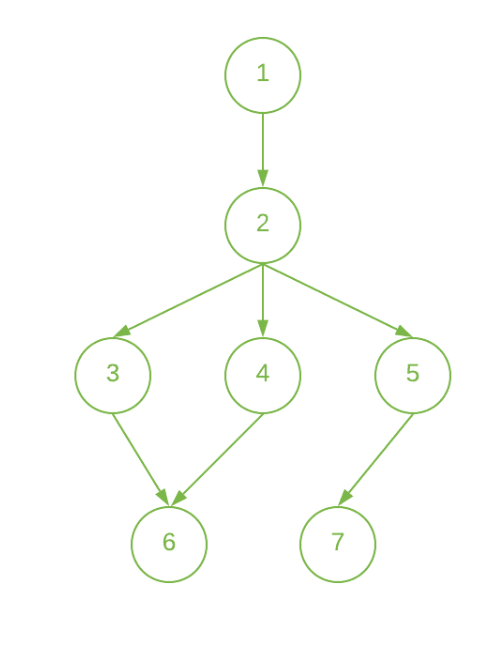GeeksforGeeks App
Open AppBrowser
Continue

# Minimum time taken by each job to be completed given by a Directed Acyclic Graph

Given a Directed Acyclic Graph having V vertices and E edges, where each edge {U, V} represents the Jobs U and V such that Job V can only be started only after completion of Job U. The task is to determine the minimum time taken by each job to be completed where each Job takes unit time to get completed.

Examples:

Input: N = 10, E = 13, Below is the given graph:Output: 1 1 2 2 2 3 4 5 2 6
Explanation:
Start the jobs 1 and 2 at the beginning and complete them at 1 unit of time.
Since, jobs 3, 4, 5, and 9 have the only dependency on one job (i.e 1st job for jobs 3, 4, and 5 and 2nd job for job 9). So, we can start these jobs at 1st unit of time and complete these at 2nd unit of time after the completion of the dependent Job.
Similarly,
Job 6 can only be done after 3rd and 4th jobs are done. So, start it at 2nd unit of time and complete it at 3rd unit of time.
Job 7 can only be done after job 6 is done. So, you can start it at 3rd unit of time and complete it at 4th unit of time.
Job 8 can only be done after 4th, 5th, and 7th jobs are done. So, start it at 4th unit of time and complete it at 5th unit of time.
Job 10 can only be done after the 8th job is done. So, start it at 5th unit of time and complete it at 6th unit of time.

Input: N = 7, E = 7, Below is the given graph:Output: 1 2 3 3 3 4 4
Explanation:
Start the Job 1 at the beginning and complete it at 1st unit of time.
The job 2 can only be done after 1st Job is done. So, start it at 1st unit of time and complete it at 2nd unit of time.
Since, Job 3, 4, and 5 have the only dependency on 2nd Job. So, start these jobs at 2nd unit of time and complete these at 3rd unit of time.
The Job 6 can only be done after the 3rd and 4th Job is done. So, start it at 3rd unit of time and complete it at 4th unit of time.
The Job 7 can only be done after the 5th Job is done. So, start it at 3rd hour and complete it at 4th unit of time.

Approach: The job can be started only if all the jobs that are prerequisites of the job that are done. Therefore, the idea is to use Topological Sort for the given network. Below are the steps:

1. Finish the jobs that are not dependent on any other job.
2. Create an array inDegree[] to store the count of the dependent node for each node in the given network.
3. Initialize a queue and push all the vertex whose inDegree[] is 0.
4. Initialize the timer to 1 and store the current queue size(say size) and do the following:
• Pop the node from the queue until the size is 0 and update the finishing time of this node to the timer.
• While popping the node(say node U) from the queue decrement the inDegree of every node connected to it.
• If inDegree of any node is 0 in the above step then insert that node in the queue.
• Increment the timer after all the above steps.
5. Print the finishing time of all the nodes after we traverse every node in the above step.

Below is the implementation of the above approach:

## C++

 `// C++ program for the above approach``#include ``using` `namespace` `std;``#define maxN 100000` `// Adjacency List to store the graph``vector<``int``> graph[maxN];` `// Array to store the in-degree of node``int` `indegree[maxN];` `// Array to store the time in which``// the job i can be done``int` `job[maxN];` `// Function to add directed edge``// between two vertices``void` `addEdge(``int` `u, ``int` `v)``{``    ``// Insert edge from u to v``    ``graph[u].push_back(v);` `    ``// Increasing the indegree``    ``// of vertex v``    ``indegree[v]++;``}` `// Function to find the minimum time``// needed by each node to get the task``void` `printOrder(``int` `n, ``int` `m)``{``    ``// Find the topo sort order``    ``// using the indegree approach` `    ``// Queue to store the``    ``// nodes while processing``    ``queue<``int``> q;` `    ``// Pushing all the vertex in the``    ``// queue whose in-degree is 0` `    ``// Update the time of the jobs``    ``// who don't require any job to``    ``// be completed before this job``    ``for` `(``int` `i = 1; i <= n; i++) {``        ``if` `(indegree[i] == 0) {``            ``q.push(i);``            ``job[i] = 1;``        ``}``    ``}` `    ``// Iterate until queue is empty``    ``while` `(!q.empty()) {` `        ``// Get front element of queue``        ``int` `cur = q.front();` `        ``// Pop the front element``        ``q.pop();` `        ``for` `(``int` `adj : graph[cur]) {` `            ``// Decrease in-degree of``            ``// the current node``            ``indegree[adj]--;` `            ``// Push its adjacent elements``            ``if` `(indegree[adj] == 0) {``                ``job[adj] = job[cur] + 1;``                ``q.push(adj);``            ``}``        ``}``    ``}` `    ``// Print the time to complete``    ``// the job``    ``for` `(``int` `i = 1; i <= n; i++)``        ``cout << job[i] << ``" "``;``    ``cout << ``"\n"``;``}` `// Driver Code``int` `main()``{``    ``// Given Nodes N and edges M``    ``int` `n, m;``    ``n = 10;``    ``m = 13;` `    ``// Given Directed Edges of graph``    ``addEdge(1, 3);``    ``addEdge(1, 4);``    ``addEdge(1, 5);``    ``addEdge(2, 3);``    ``addEdge(2, 8);``    ``addEdge(2, 9);``    ``addEdge(3, 6);``    ``addEdge(4, 6);``    ``addEdge(4, 8);``    ``addEdge(5, 8);``    ``addEdge(6, 7);``    ``addEdge(7, 8);``    ``addEdge(8, 10);` `    ``// Function Call``    ``printOrder(n, m);``    ``return` `0;``}`

## Java

 `// Java program for the above approach``import` `java.util.*;` `class` `GFG{``    ` `static` `final` `int` `maxN = ``100000``;` `// Adjacency List to store the graph``@SuppressWarnings``(``"unchecked"``)``static` `Vector []graph = ``new` `Vector[maxN];` `// Array to store the in-degree of node``static` `int` `[]indegree = ``new` `int``[maxN];` `// Array to store the time in which``// the job i can be done``static` `int` `[]job = ``new` `int``[maxN];` `// Function to add directed edge``// between two vertices``static` `void` `addEdge(``int` `u, ``int` `v)``{``    ` `    ``// Insert edge from u to v``    ``graph[u].add(v);` `    ``// Increasing the indegree``    ``// of vertex v``    ``indegree[v]++;``}` `// Function to find the minimum time``// needed by each node to get the task``static` `void` `printOrder(``int` `n, ``int` `m)``{``    ` `    ``// Find the topo sort order``    ``// using the indegree approach` `    ``// Queue to store the``    ``// nodes while processing``    ``Queue q = ``new` `LinkedList<>();``    ` `    ``// Pushing all the vertex in the``    ``// queue whose in-degree is 0` `    ``// Update the time of the jobs``    ``// who don't require any job to``    ``// be completed before this job``    ``for``(``int` `i = ``1``; i <= n; i++)``    ``{``        ``if` `(indegree[i] == ``0``)``        ``{``            ``q.add(i);``            ``job[i] = ``1``;``        ``}``    ``}` `    ``// Iterate until queue is empty``    ``while` `(!q.isEmpty())``    ``{` `        ``// Get front element of queue``        ``int` `cur = q.peek();` `        ``// Pop the front element``        ``q.remove();` `        ``for``(``int` `adj : graph[cur])``        ``{``            ` `            ``// Decrease in-degree of``            ``// the current node``            ``indegree[adj]--;` `            ``// Push its adjacent elements``            ``if` `(indegree[adj] == ``0``){``                ``job[adj] = ``1` `+ job[cur];``                ``q.add(adj);``            ``}``        ``}``    ``}` `    ``// Print the time to complete``    ``// the job``    ``for``(``int` `i = ``1``; i <= n; i++)``        ``System.out.print(job[i] + ``" "``);``    ``System.out.print(``"\n"``);``}` `// Driver Code``public` `static` `void` `main(String[] args)``{``    ` `    ``// Given Nodes N and edges M``    ``int` `n, m;``    ``n = ``10``;``    ``m = ``13``;``    ` `    ``for``(``int` `i = ``0``; i < graph.length; i++)``        ``graph[i] = ``new` `Vector();``        ` `    ``// Given directed edges of graph``    ``addEdge(``1``, ``3``);``    ``addEdge(``1``, ``4``);``    ``addEdge(``1``, ``5``);``    ``addEdge(``2``, ``3``);``    ``addEdge(``2``, ``8``);``    ``addEdge(``2``, ``9``);``    ``addEdge(``3``, ``6``);``    ``addEdge(``4``, ``6``);``    ``addEdge(``4``, ``8``);``    ``addEdge(``5``, ``8``);``    ``addEdge(``6``, ``7``);``    ``addEdge(``7``, ``8``);``    ``addEdge(``8``, ``10``);` `    ``// Function call``    ``printOrder(n, m);``}``}` `// This code is contributed by Amit Katiyar`

## Python3

 `# Python3 program for the above approach``from` `collections ``import` `defaultdict` `# Class to represent a graph``class` `Graph:``    ` `    ``def` `__init__(``self``, vertices, edges):``        ` `        ``# Dictionary containing adjacency List``        ``self``.graph ``=` `defaultdict(``list``)``        ` `        ``# No. of vertices``        ``self``.n ``=` `vertices ``        ` `        ``# No. of edges``        ``self``.m ``=` `edges ``        ` `    ``# Function to add an edge to graph``    ``def` `addEdge(``self``, u, v):``        ``self``.graph[u].append(v)``    ` `    ``# Function to find the minimum time``    ``# needed by each node to get the task``    ``def` `printOrder(``self``, n, m):``      ` `        ``# Create a vector to store indegrees of all``        ``# vertices. Initialize all indegrees as 0.``        ``indegree ``=` `[``0``] ``*` `(``self``.n ``+` `1``)``        ` `        ``# Traverse adjacency lists to fill indegrees``        ``# of vertices. This step takes O(V + E) time``        ``for` `i ``in` `self``.graph:``            ``for` `j ``in` `self``.graph[i]:``                ``indegree[j] ``+``=` `1``                ` `        ``# Array to store the time in which``        ``# the job i can be done``        ``job ``=` `[``0``] ``*` `(``self``.n ``+` `1``)``        ` `        ``# Create an queue and enqueue all``        ``# vertices with indegree 0``        ``q ``=` `[]``        ` `        ``# Update the time of the jobs``        ``# who don't require any job to``        ``# be completed before this job``        ``for` `i ``in` `range``(``1``, ``self``.n ``+` `1``):``            ``if` `indegree[i] ``=``=` `0``:``                ``q.append(i)``                ``job[i] ``=` `1``                ` `        ``# Iterate until queue is empty``        ``while` `q:``            ` `            ``# Get front element of queue``            ``cur ``=` `q.pop(``0``)``            ` `            ``for` `adj ``in` `self``.graph[cur]:``                ` `                ``# Decrease in-degree of``                ``# the current node``                ``indegree[adj] ``-``=` `1``              ` `                ``# Push its adjacent elements``                ``if` `(indegree[adj] ``=``=` `0``):``                    ``job[adj] ``=`  `1` `+` `job[cur]``                    ``q.append(adj)``                    ` `        ``# Print the time to complete``        ``# the job``        ``for` `i ``in` `range``(``1``, n ``+` `1``):``            ``print``(job[i], end ``=` `" "``)``            ` `        ``print``()` `# Driver Code` `# Given Nodes N and edges M``n ``=` `10``m ``=` `13` `g ``=` `Graph(n, m)` `# Given Directed Edges of graph``g.addEdge(``1``, ``3``)``g.addEdge(``1``, ``4``)``g.addEdge(``1``, ``5``)``g.addEdge(``2``, ``3``)``g.addEdge(``2``, ``8``)``g.addEdge(``2``, ``9``)``g.addEdge(``3``, ``6``)``g.addEdge(``4``, ``6``)``g.addEdge(``4``, ``8``)``g.addEdge(``5``, ``8``)``g.addEdge(``6``, ``7``)``g.addEdge(``7``, ``8``)``g.addEdge(``8``, ``10``)` `# Function Call``g.printOrder(n, m)` `# This code is contributed by Aanchal Tiwari`

## C#

 `// C# program for the above approach``using` `System;``using` `System.Collections.Generic;` `class` `GFG{``    ` `static` `readonly` `int` `maxN = 100000;` `// Adjacency List to store the graph``static` `List<``int``> []graph = ``new` `List<``int``>[maxN];` `// Array to store the in-degree of node``static` `int` `[]indegree = ``new` `int``[maxN];` `// Array to store the time in which``// the job i can be done``static` `int` `[]job = ``new` `int``[maxN];` `// Function to add directed edge``// between two vertices``static` `void` `addEdge(``int` `u, ``int` `v)``{``    ` `    ``// Insert edge from u to v``    ``graph[u].Add(v);` `    ``// Increasing the indegree``    ``// of vertex v``    ``indegree[v]++;``}` `// Function to find the minimum time``// needed by each node to get the task``static` `void` `printOrder(``int` `n, ``int` `m)``{``    ` `    ``// Find the topo sort order``    ``// using the indegree approach` `    ``// Queue to store the``    ``// nodes while processing``    ``Queue<``int``> q = ``new` `Queue<``int``>();``    ` `    ``// Pushing all the vertex in the``    ``// queue whose in-degree is 0` `    ``// Update the time of the jobs``    ``// who don't require any job to``    ``// be completed before this job``    ``for``(``int` `i = 1; i <= n; i++)``    ``{``        ``if` `(indegree[i] == 0)``        ``{``            ``q.Enqueue(i);``            ``job[i] = 1;``        ``}``    ``}` `    ``// Iterate until queue is empty``    ``while` `(q.Count != 0)``    ``{` `        ``// Get front element of queue``        ``int` `cur = q.Peek();` `        ``// Pop the front element``        ``q.Dequeue();` `        ``foreach``(``int` `adj ``in` `graph[cur])``        ``{``            ` `            ``// Decrease in-degree of``            ``// the current node``            ``indegree[adj]--;` `            ``// Push its adjacent elements``            ``if` `(indegree[adj] == 0){``                ``job[adj] = 1 + job[cur];``                ``q.Enqueue(adj);``            ``}``        ``}``    ``}` `    ``// Print the time to complete``    ``// the job``    ``for``(``int` `i = 1; i <= n; i++)``        ``Console.Write(job[i] + ``" "``);``        ` `    ``Console.Write(``"\n"``);``}` `// Driver Code``public` `static` `void` `Main(String[] args)``{``    ` `    ``// Given Nodes N and edges M``    ``int` `n, m;``    ``n = 10;``    ``m = 13;``    ` `    ``for``(``int` `i = 0; i < graph.Length; i++)``        ``graph[i] = ``new` `List<``int``>();``        ` `    ``// Given directed edges of graph``    ``addEdge(1, 3);``    ``addEdge(1, 4);``    ``addEdge(1, 5);``    ``addEdge(2, 3);``    ``addEdge(2, 8);``    ``addEdge(2, 9);``    ``addEdge(3, 6);``    ``addEdge(4, 6);``    ``addEdge(4, 8);``    ``addEdge(5, 8);``    ``addEdge(6, 7);``    ``addEdge(7, 8);``    ``addEdge(8, 10);` `    ``// Function call``    ``printOrder(n, m);``}``}` `// This code is contributed by Amit Katiyar`

## Javascript

 `const maxN = 100000;` `// Adjacency List to store the graph``const graph = ``new` `Array(maxN);``for` `(let i = 0; i < maxN; i++) {``    ``graph[i] = [];``}` `// Array to store the in-degree of node``const indegree = ``new` `Array(maxN).fill(0);` `// Array to store the time in which``// the job i can be done``const job = ``new` `Array(maxN).fill(0);` `// Function to add directed edge``// between two vertices``function` `addEdge(u, v) {``    ``// Insert edge from u to v``    ``graph[u].push(v);` `    ``// Increasing the indegree``    ``// of vertex v``    ``indegree[v]++;``}` `// Function to find the minimum time``// needed by each node to get the task``function` `printOrder(n, m) {``    ``// Find the topo sort order``    ``// using the indegree approach` `    ``// Queue to store the``    ``// nodes while processing``    ``const q = [];` `    ``// Pushing all the vertex in the``    ``// queue whose in-degree is 0` `    ``// Update the time of the jobs``    ``// who don't require any job to``    ``// be completed before this job``    ``for` `(let i = 1; i <= n; i++) {``        ``if` `(indegree[i] === 0) {``            ``q.push(i);``            ``job[i] = 1;``        ``}``    ``}` `    ``// Iterate until queue is empty``    ``while` `(q.length > 0) {` `        ``// Get front element of queue``        ``const cur = q.shift();` `        ``for` `(const adj of graph[cur]) {` `            ``// Decrease in-degree of``            ``// the current node``            ``indegree[adj]--;` `            ``// Push its adjacent elements``            ``if` `(indegree[adj]== 0) {``job[adj] = job[cur] + 1;``q.push(adj);``}``}``}` `// Print the time to complete``// the job``for` `(let i = 1; i <= n; i++) {``    ``console.log(job[i]);``}``}``// Driver Code``function` `main() {``// Given Nodes N and edges M``const n = 10;``const m = 13;` `// Given Directed Edges of graph``addEdge(1, 3);``addEdge(1, 4);``addEdge(1, 5);``addEdge(2, 3);``addEdge(2, 8);``addEdge(2, 9);``addEdge(3, 6);``addEdge(4, 6);``addEdge(4, 8);``addEdge(5, 8);``addEdge(6, 7);``addEdge(7, 8);``addEdge(8, 10);` `// Function Call``printOrder(n, m);``}` `main();``// this code is contributed by devendrasalunke`

Output:

`1 1 2 2 2 3 4 5 2 6`

Time Complexity: O(V+E), where V is the number of nodes and E is the number of edges.
Auxiliary Space: O(V)

My Personal Notes arrow_drop_up